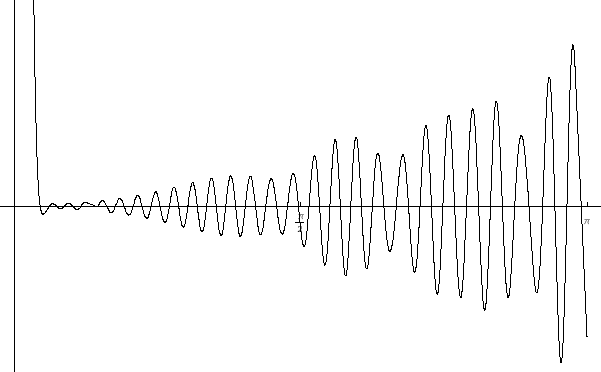This is the graph of the polynomial t50(z) of a sequence of cosine polynomials {tn(z)} which aproximates locally uniformly the Riemann x function (in Titchmarsh's book on the Riemann z function x is denoted by X). As you may observe our "Christmas tree" logo is a part of the above graph. Even more important observation is that ALL the zeros of t50(z) are real. Is the same true for every natural n?!?